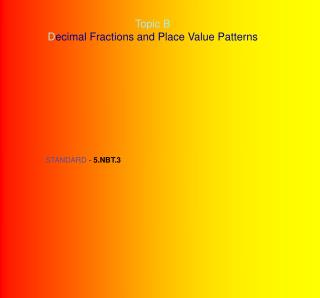DownloadDownload PresentationTopic B D ecimal Fractions and Place Value Patterns

# Topic B D ecimal Fractions and Place Value Patterns

Download Presentation## Topic B D ecimal Fractions and Place Value Patterns

- - - - - - - - - - - - - - - - - - - - - - - - - - - E N D - - - - - - - - - - - - - - - - - - - - - - - - - - -
##### Presentation Transcript

1. Topic B Decimal Fractions and Place Value Patterns STANDARD - 5.NBT.3

2. Grade 5 Module 1 Lesson 5 Objective: Name decimal fractions in expanded,  unit and word form by applying place  value reasoning.

3. MATERIALS NEEDED FOR THIS LESSON Student Student Pages Place Value Chart Personal White Boards Sprint A & B Problem Set Exit Ticket Homework Teacher Teacher Pages

4. Fluency Practice(12 minutes)

5. Multiply and Divide by 10, 100, 1000 8 minutes 4.NBT.1 SPRINT A 1 minutes Do as many problems as you can. Do your personal best. Take your mark! Get set! THINK!

6. Multiply and Divide by 10, 100, 1000 4.NBT.1 SPRINT B 1 minutes Do as many problems as you can. Do your personal best. Take your mark! Get set! THINK!

7. Multiply and Divide by Exponents 2 minutes 5.NBT.2 Write 54 tenths as a decimal

8. Write 0.6 x 102

9. Write 0.6 102

10. Write 2.784 x 103

11. Write 6583 103

12. Multiplying Metric Units 2 minutes 5.MD.1 3 m = _____ cm Show on your place value chart. How many centimeters are in 1 meter? How many centimeters in 3 meters? Show on your place value chart.

13. 7 kg = _____ g 7000 ml = _____ l 7500 m = _____ km _____ m 8350 g = _____ kg _____ g

14. Application Problem (8 minutes) Jordan measures a desk at 200 cm. James  measures the same desk in milliliters, and Amy  measures the same desk in meters. What is  James measurement in milliliters? What is  Amy's measurement in meters? Show your  thinking using a place value mat or equation  using place value mat or an equation with  exponents.

15. Concept Development (30 minutes)

16. Opener three thousand forty seven standard form: _____________________

17. Problem 1 Represent in standard and unit form. one thousandths one thousandth = 0.001 = 1 1000 1 = 1 x 1 1000  1000 0.001 = 1 x 0.001 1 thousandths notes Write in digits on place value chart. standard form: ________________________ as a fraction: ________________________ expanded form: using fractions ________________________ using decimals ________________________

18. If you had 3 copies of 1 thousandths, how many thousandths is that? three thousandth = 0.003 = 3 1000 3 = 3 x 1 1000  1000 0.003 = 3 x 0.001 3 thousandths notes Write in digits on place value chart. standard form: ________________________ as a fraction: ________________________ expanded form: using fractions ________________________ using decimals ________________________

19. Problem 2 represent a number thousandths in standard form and unit form thirteen thousandth = 0.013 = 13 1000 13 = 1000  0.013 x 0.01+ 3 x 0.001 1 hundredth 3 thousandths 13 thousandths notes thirteen thousandths standard form: ________________________ expanded form: using fractions ________________________ using decimals ________________________

20. two hundred seventy three thousandths standard form: ________________________ expanded form: using fractions ________________________ using decimals ________________________ are these the same? 2 tenths 73 thousandths 273 thousandths 27 hundredths 3 thousandths ???

21. one and six hundred eight thousandths standard form: ________________________ expanded form: using fractions ________________________ using decimals ________________________

22. Problem 3 Twenty-five and four hundred thirteen thousandths notes represent a number in word, expanded and unit form 413 1000 25.413 25 = 25.413 1 10 1 100 413 1000 1 1000 25 = 2 x 10 + 5 x 1 + 4 x + 1 x + 3 x word form:__________________________ decimal unit form: ____________________ other unit forms: _____________________ 25.413 = 2 x 10 + 5 x 1 + 4 x 0.1 + 1 x 0.01 + 3 x 0.001 2 tens 5 ones 4 tenths 1 hundredths 3 thousandths 25 ones 413 thousandths

23. 12.04 word form:__________________________ decimal unit form: ____________________ other unit forms: _____________________

24. 9.495 word form:__________________________ decimal unit form: ____________________ other unit forms: _____________________

25. Problem 4 write the standard, expanded and unit forms write the expanded and unit forms  of each number 404 1000 four hundred four thousandths = = 0.404 404 1000 1 10 1 1000 = 4 x + 4 x four hundred four thousandths four hundred and four thousandths notes 4 1000 four hundred and four thousandths = 400 = 400.004 0.404 = 4 x 0.1 + 4 x 0.001 4 tenths 4 thousandths 4 1000 1 1000 400 = 4 x 100 + 4 x 400.004 = 4 x 100 + 4 x 0.001 notes 2

26. write the expanded and unit forms  of each number two hundred two thousandths nine hundred and nine tenths

27. Problem Set (10 minutes) Do Your Personal Best (Complete independently!)

28. Student Debrief (10 minutes) When might expanded form be useful as a  calculation tool? Which tasks in #1 are alike? Why? What is the purpose of writing a decimal  number in expanded form using fractions?  What was the objective of our lesson today? How is expanded form related to the standard  form of a number? Compare your answers to #1 c and d. What is  the importance of the word and when naming  decimals in standard form?

29. EXIT TICKET (3 minutes) Complete the exit ticket and turn in...

30. HOMEWORK